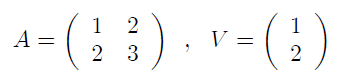## Friday, September 18, 2020

Solution Exercise 550 : numpy matrix product

#### Exercise 550

Given a square matrix A and a vector V:Give the Python code numpy which calculates the product AV (the matrix product and not the term to term product)

#### Solution

``````import numpy as np
A = np.array([[1,2],
[2,3]])
V =  np.array([1,2])
print(np.dot(A,V))``````

Younes Derfoufi
my-courses.net

## Python Exercises With Solutions

1. Python Arithmetic Exercises
2. Equations & System Of Equations
3. Polynomials and Functions
4. Python Arithmetic Exercises
5. Numpy, Matplolib, Sympy , Scipy - Exercises
6. Python Arithmetic Exercises

Younes Derfoufi

## Sample Text